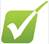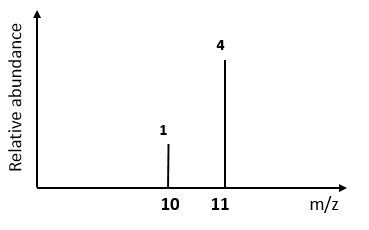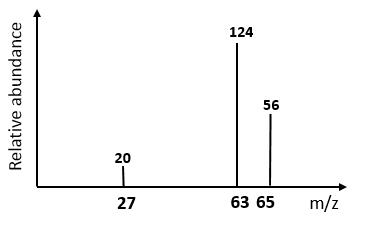Mass spectroscopy and Ar calculations## 1)   A sample of sulphur has three isotopes: sulphur-32, sulphur-33 and sulphur-34. Calculate the relative atomic mass from the data below:  Give your answer to the appropriate number of decimals places.

 percentage abundance 95.02 0.76 4.22 Isotopic mass 32 33 34

2)  Boron has 2 isotopes and the mass spectrum is shown below.  Calculate its relative atomic mass:3)   Lithium has two naturally occurring isotopes 6Li and 7Li. Calculate the percentage abundance of each isotopes given that the Ar of Lithium is 6.9.  Show your working.

4)  A specific alloy of bronze has been developed for use in aircraft due to its high strength and resistance to corrosion.

It is an alloy of two metals and ts mass spectrum is shown below. Identify the two metals in this bronze and explain why there are three peaks.5)  For the above question, calculate the relative atomic mass of the element with Isotopes.

## 6)   A sample of magnesium has three isotopes: magnesium-24, magnesium -25 and magnesium -26. Given that the relative atomic mass of magnesium is 24.3, calculate the relative abundance for magnesium 24 from the data below:

 Relative abundance 100 110 Isotopic mass 24 25 26

7)  Sketch the mass spectrum you would likely see for Chlorine molecules given the following data:

 Relative abundance 3 1 Isotopic mass 35 37

8)  Mass spectroscopy is a technique used to determine relative abundancies of isotopes of elements.

a)  Briefly explain the stages in mass spectroscopy:

b)  Write an equation showing the ionisation stage, use M to represent an atom:

c)  Write an equation to show what happens at the detector.